# A Minimization Model Example[Page 47 ( continued )]

As mentioned at the beginning of this chapter, there are two types of linear programming problems: maximization problems (like the Beaver Creek Pottery Company example) and minimization problems. A minimization problem is formulated the same basic way as a maximization problem, except for a few minor differences. The following sample problem will demonstrate the formulation of a minimization model.

A farmer is preparing to plant a crop in the spring and needs to fertilize a field. There are two brands of fertilizer to choose from, Super-gro and Crop-quick. Each brand yields a specific amount of nitrogen and phosphate per bag, as follows :

Chemical Contribution

Brand

N ITROGEN ( LB./BAG )

P HOSPHATE ( LB./BAG )

Super-gro

2

4

Crop-quick

4

3

The farmer's field requires at least 16 pounds of nitrogen and 24 pounds of phosphate. Super-gro costs \$6 per bag, and Crop-quick costs \$3. The farmer wants to know how many bags of each brand to purchase in order to minimize the total cost of fertilizing. This scenario is illustrated in Figure 2.15.

The steps in the linear programming model formulation process are summarized as follows:

## Summary of LP Model Formulation Steps

 Step  1. Define the decision variables How many bags of Super-gro and Crop-quick to buy Step  2. Define the objective function Minimize cost Step  3. Define the constraints The field requirements for nitrogen and phosphate

#### Decision Variables

This problem contains two decision variables, representing the number of bags of each brand of fertilizer to purchase:

x 1 = bags of Super-gro

x 2 = bags of Crop-quick

[Page 48]
##### Figure 2.15. Fertilizing farmer's field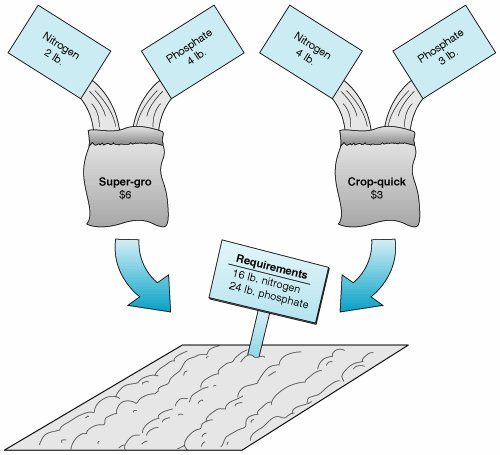#### The Objective Function

The farmer's objective is to minimize the total cost of fertilizing. The total cost is the sum of the individual costs of each type of fertilizer purchased. The objective function that represents total cost is expressed as

minimize Z = \$6 x 1 + 3 x 2

where

\$6 x 1 = cost of bags of Super-gro

\$3 x 2 = cost of bags of Crop-quick

#### Model Constraints

The requirements for nitrogen and phosphate represent the constraints of the model. Each bag of fertilizer contributes a number of pounds of nitrogen and phosphate to the field. The constraint for nitrogen is

2 x 1 + 4 x 2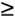16 lb.

where

2 x 1 = the nitrogen contribution (lb.) per bag of Super-gro

4 x 2 = the nitrogen contribution (lb.) per bag of Crop-quick

Rather than a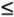(less than or equal to) inequality, as used in the Beaver Creek Pottery Company model, this constraint requires a(greater than or equal to) inequality. This is because the nitrogen content for the field is a minimum requirement specifying that at least 16 pounds of nitrogen be deposited on the farmers field. If a minimum cost solution results in more than 16 pounds of nitrogen on the field, that is acceptable; however, the amount cannot be less than 16 pounds.

[Page 49]

The constraint for phosphate is constructed like the constraint for nitrogen:

4 x 1 + 3 x 224 lb.

With this example we have shown two of the three types of linear programming model constraints,and. The third type is an exact equality, =. This type specifies that a constraint requirement must be exact. For example, if the farmer had said that the phosphate requirement for the field was exactly 24 pounds, the constraint would have been

The three types of linear programming constraints are, =, and.

4 x 1 + 3 x 2 = 24 lb.

As in our maximization model, there are also nonnegativity constraints in this problem to indicate that negative bags of fertilizer cannot be purchased:

x 1 , x 2The complete model formulation for this minimization problem is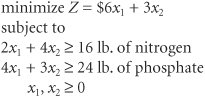#### Graphical Solution of a Minimization Model

We follow the same basic steps in the graphical solution of a minimization model as in a maximization model. The fertilizer example will be used to demonstrate the graphical solution of a minimization model.

The first step is to graph the equations of the two model constraints, as shown in Figure 2.16. Next, the feasible solution area is chosen , to reflect theinequalities in the constraints, as shown in Figure 2.17.

##### Figure 2.16. Constraint lines for fertilizer model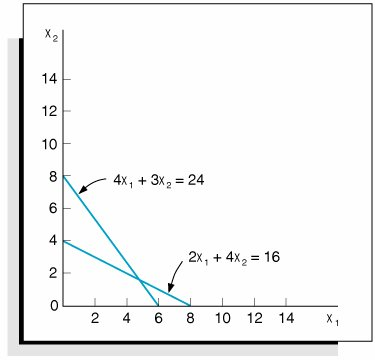[Page 50]
##### Figure 2.17. Feasible solution area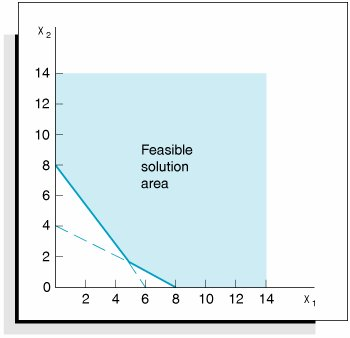After the feasible solution area has been determined, the second step in the graphical solution approach is to locate the optimal point. Recall that in a maximization problem, the optimal solution is on the boundary of the feasible solution area that contains the point(s) farthest from the origin. The optimal solution point in a minimization problem is also on the boundary of the feasible solution area; however, the boundary contains the point(s) closest to the origin (zero being the lowest cost possible).

The optimal solution of a minimization problem is at the extreme point closest to the origin .

As in a maximization problem, the optimal solution is located at one of the extreme points of the boundary. In this case the corner points represent extremities in the boundary of the feasible solution area that are closest to the origin. Figure 2.18 shows the three corner points A , B , and C and the objective function line.

As the objective function edges toward the origin, the last point it touches in the feasible solution area is A . In other words, point A is the closest the objective function can get to the origin without encompassing infeasible points. Thus, it corresponds to the lowest cost that can be attained.

##### Figure 2.18. The optimal solution point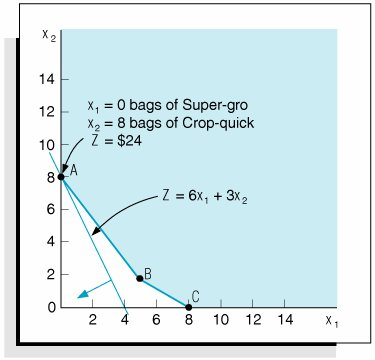[Page 51]

## Management Science Application: Determining Optimal Fertilizer Mixes at Soquimich (South America)

Soquimich, a Chilean fertilizer manufacturer, is the leading producer and distributor of specialty fertilizers in the world, with revenues of almost US\$0.5 billion in more than 80 countries . Soquimich produces four main specialty fertilizers and more than 200 fertilizer blends, depending on the needs of its customers. Farmers want the company to quickly recommend optimal fertilizer blends that will provide the appropriate quantity of ingredients for their particular crop at the lowest possible cost. A farmer will provide a company sales representative with information about previous crop yields and his or her target yields and then company representatives will visit the farm to obtain soil samples, which are analyzed in the company labs. A report is generated, which indicates the soil requirements for nutrients, including nitrogen, phosphorus, potassium, boron, magnesium, sulfur, and zinc. Given these soil requirements, company experts determine an optimal fertilizer blend, using a linear programming model that includes constraints for the nutrient quantities required by the soil (for a particular crop) and an objective function that minimizes production costs. Previously the company determined fertilizer blend recommendations by using a time-consuming manual procedure conducted by experts. The linear programming model enables the company to provide accurate, quick, low-cost (discounted) estimates to its customers, which has helped the company gain new customers and increase its market share.Source: A. M. Angel, L. A. Taladriz, and R. Weber. "Soquimich Uses a System Based on Mixed-Integer Linear Programming and Expert Systems to Improve Customer Service," Interfaces 33, no. 4 (JulyAugust 2003): 4152.

The final step in the graphical solution approach is to solve for the values of x 1 and x 2 at point A . Because point A is on the x 2 axis, x 1 = 0; thus,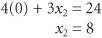Given that the optimal solution is x 1 = 0, x 2 = 8, the minimum cost, Z , is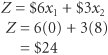This means the farmer should not purchase any Super-gro but, instead, should purchase eight bags of Crop-quick, at a total cost of \$24.

#### Surplus Variables

Greater than or equal to constraints cannot be converted to equations by adding slack variables, as withconstraints. Recall our fertilizer model, formulated as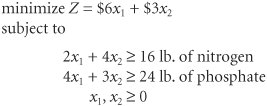[Page 52]

where

x 1 = bags of Super-gro fertilizer

x 2 = bags of Crop-quick fertilizer

Z = farmer's total cost (\$) of purchasing fertilizer

Because this problem hasconstraints as opposed to theconstraints of the Beaver Creek Pottery Company maximization example, the constraints are converted to equations a little differently.

A surplus variable is subtracted from aconstraint to convert it to an equation (=) .

Instead of adding a slack variable with aconstraint, we subtract a surplus variable . Whereas a slack variable is added and reflects unused resources, a surplus variable is subtracted and reflects the excess above a minimum resource requirement level. Like a slack variable, a surplus variable is represented symbolically by s 1 and must be nonnegative.

For the nitrogen constraint, the subtraction of a surplus variable gives

A surplus variable represents an excess above a constraint requirement level .

2 x 1 + 4 x 2 s 1 = 16

The surplus variable s 1 transforms the nitrogen constraint into an equation.

As an example, consider the hypothetical solution

x 1 = 0

x 2 = 10

Substituting these values into the previous equation yields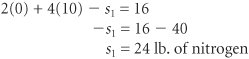In this equation s 1 can be interpreted as the extra amount of nitrogen above the minimum requirement of 16 pounds that would be obtained by purchasing 10 bags of Crop-quick fertilizer.

In a similar manner, the constraint for phosphate is converted to an equation by subtracting a surplus variable, s 2 :

4 x 1 + 3 x 2 s 2 = 24

##### Figure 2.19. Graph of the fertilizer example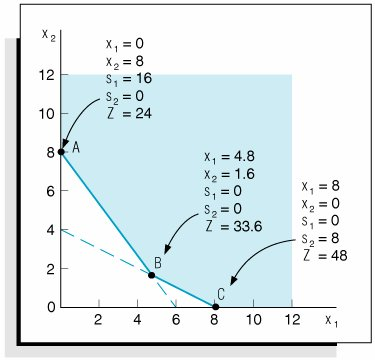[Page 53]

As is the case with slack variables, surplus variables contribute nothing to the overall cost of a model. For example, putting additional nitrogen or phosphate on the field will not affect the farmer's cost; the only thing affecting cost is the number of bags of fertilizer purchased. As such, the standard form of this linear programming model is summarized as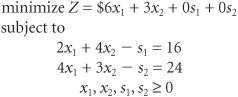Figure 2.19 shows the graphical solutions for our example, with surplus variables included at each solution point.Introduction to Management Science (10th Edition)
ISBN: 0136064361
EAN: 2147483647
Year: 2006
Pages: 358

Similar book on Amazon

flylib.com © 2008-2017.
If you may any questions please contact us: flylib@qtcs.net